Home PHYSICS TOPIC 3: LIGHT | PHYSICS FORM 3

# TOPIC 3: LIGHT | PHYSICS FORM 3

79
0
SHARE## TOPIC 3: LIGHT | PHYSICS FORM 3

Light is a form of energy which controls the sense of vision.

Difference between Concave and Convex Mirrors
Distinguish between concave and convex mirrors
Concave mirror is a spherical mirror whose reflecting surface is curved inwards. A Good example is the driving mirror of a car.
Convex mirror is a spherical mirror whose reflective surface is curved outwards. A good example of a convex mirror is a shaving mirror.
General demonstrations of convex and concave mirrors (curved mirrors: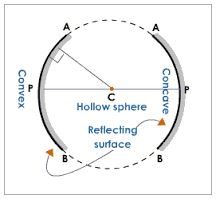The Terms Principle, Axis, Pole, Principle Focus and Radius of Curvature as Applied to Curved Mirrors
Explain the terms principle, axis, pole, principle focus and radius of curvature as applied to curved mirrors
Terms used in studying curved mirrors
• Centre of curvature (C):the centre of the sphere of which a mirror is a part of.
• Radius of curvature (R): the radius of sphere of which a mirror is a part of.
• Pole (P): the central point of the reflecting surface of spherical mirror (curved or convex mirror).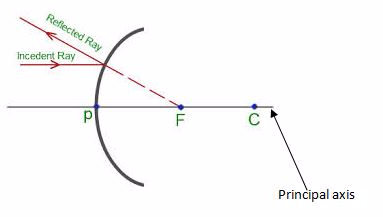• Principal axis:the straight line joining the centre of curvature (C) and the pole (P).
• Principal focus (F):the point o the principal axis where light rays tend to intersect. This point is between centre of curvature and the pole.
• Principal axis:the straight line joining the centre of curvature (C) and the pole (P).
• Principal focus (F):the point on the principal axis where light rays tend to intersect. This point is between centre of curvature and the pole.
The Images Formed by a Curved Mirror
Locate the images formed by a curved mirror
Case (1)
When
a beam of light parallel and very close to the principal axis, CL, is
reflected from a concave mirror, it converges to a point, F, on the
principal axis called the principal focus.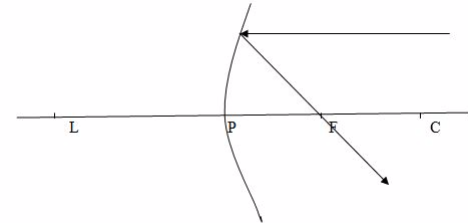Case 2
When a ray passes through the principal focus, F, it is reflected parallel to the principal axis.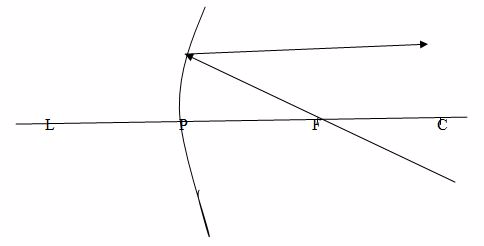Case 3
When
a ray passes through the centre of curvature, C, which therefore
strikes the mirror at normal incidence, it is reflected back along its
original path.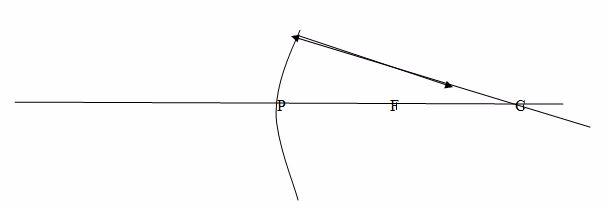Note: Concave mirrors have a real focus because light passes through the focus.
The formation of images by concave mirror tends to change as the position of object changes.
Case 1: Image (I) formed by a concave mirror when the object is beyond C.Properties of images formed:
1. The image is between C and F
2. The image is smaller than the object
3. The image is inverted (upside down)
4. The image is real
Case 2: The object is placed at CProperties of image
1. The image is formed at C
2. The image has the same size as object
3. The image is inverted (upside down)
4. The image is real.
Case 3: The object is placed between C and FProperties of image formed
1. The image is real
2. The image is large than object
3. The image is formed beyond C
4. The image is inverted (upside down)
5. The image is real
6. The image is large than object
7. The image is formed beyond
8. The image is inverted (upside down)
Case 4:The object is placed at FProperties of image:
1. The image is formed at infinity (x)
2. The image is formed beyond C
3. The image is large than object
4. The image is Real
Case 5:The object is placed between F and P.Properties of image formed:
1. The image is virtual
2. The images is upright
3. The image is formed behind the mirror
4. The image is large than the object
Formation of images in a convex mirror:
Obviously,there isonly one kind of image formed when an object is placed at any position.
Properties of image formed by convex mirror:
1. the image is virtual
2. the image is upright
3. The image is smaller than object (diminished)
4. The image is formed behind the mirror.
Example 1
An
object 2cm long is erected 8cm infront of a concave mirror of radius of
curvature 10cm. By using a scale drawing, determine the position, size
and nature of image formed.
Data given
• Height of object, Ho = 2cm
• Object distance, U= 8cm
• Radius of curvature, r = 10cm
• Focal length,f =8cm
• Choose suitable scale.
• Say 1cm represents 5cm
From this scale then
• Height of object, Ho = 2cm
• Object distance, U= 2cm
• Focal length, F = 2.5cm
Thus,
Image distance, V = X
Image Height, H1=Y
The Focal Length of a Concave Mirror
Determine practically the focal length of a concave mirror
Focal length (f) is the distance between the principal focus and the pole.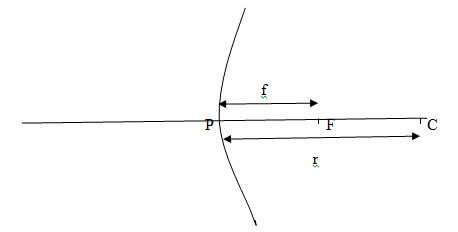Convex and Concave Mirrors in Daily Life
Use Convex and concave mirrors in daily life
Curved mirrors are used as:
1. Driving mirrors
2. Shaving mirrors
3. Reflectors
Question Time 1
Why is convex mirror used as driving mirror?
The convex mirror is used as driving mirror because it provides the wider field of view.
Question Time 2
Why concave mirror used as shaving mirror?
Concave mirrors are used as shaving mirrors because they form an enlarged image when held close up.